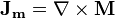# Sphere of magnetic material in an applied field

• dave4000

## Homework Statement

Consider a sphere of magnetic material with radius R and magnetisation M in an applied field $$B_{applied}$$. Find an expression for:

a) the total current flowing around the surface of the sphere;
b) the net field at the centre of the sphere.

## Homework Equations## The Attempt at a Solution

I am totally new to the magnetisation physics, and am having trouble getting to grips with it, any pointers on how to go about solving this?

Last edited by a moderator:
$$I=\int J.dS = \int (\nabla \times M).dS = 4\pi R^{2}M$$

Is this correct without $$B_{applied}$$? If so, what happens when $$B_{applied}$$ is present?